# Insert 4

Insert three arithmetic means between 3 and 63.

a =  18
b =  33
c =  48

### Step-by-step explanation:

$b=a+d=18+15=33$
$c=b+d=33+15=48$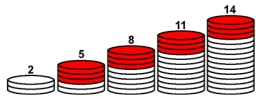Did you find an error or inaccuracy? Feel free to write us. Thank you!## Related math problems and questions:

• Insert 6Insert four harmonic means between 3/7 and 3/19
• Insert 7Insert five harmonic means between 3 and 18
• Insert 3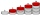Insert five arithmetic progression members between -7 and 3/2.
• Harmonic seriesInsert four members between 5/3 and 5/11 to form harmonic series (means).
• Insert AP memberInsert arithmetic means between 75 and 180.
• Insert 5Insert five harmonic means between 1/2 and 1/26
• The schoolThe school has 268 boys. Girls are 60 more than boys. How many children are there at school?
• SequenceBetween numbers 1 and 53 insert n members of the arithmetic sequence that its sum is 702.
• Geometric progression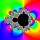Fill 4 numbers between 4 and -12500 to form geometric progression.
• Chess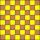How many ways can select 4 fields on a classic chessboard with 64 fields so that fields don't have the same color?
• Sum of membersWhat is the sum of the first two members of the aritmetic progression if d = -4.3 and a3 = 7.5?
• Insert into GPBetween numbers 5 and 640, insert as many numbers to form geometric progression so the sum of the numbers you entered will be 630. How many numbers must you insert?
• HP - harmonic progressionDetermine the 10th term of the harmonic progression 6,4,3,…
• Five element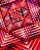The geometric sequence is given by quotient q = 1/2 and the sum of the first six members S6 = 63. Find the fifth element a5.
• Geometric sequence 4It is given geometric sequence a3 = 7 and a12 = 3. Calculate s23 (= sum of the first 23 members of the sequence).
• HP - harmonic progressionDetermine the 8th term of the harmonic progression 2, 4/3, 1,…
• AS sequenceIn an arithmetic sequence is given the difference d = -3 and a71 = 455. a) Determine the value of a62 b) Determine the sum of 71 members.